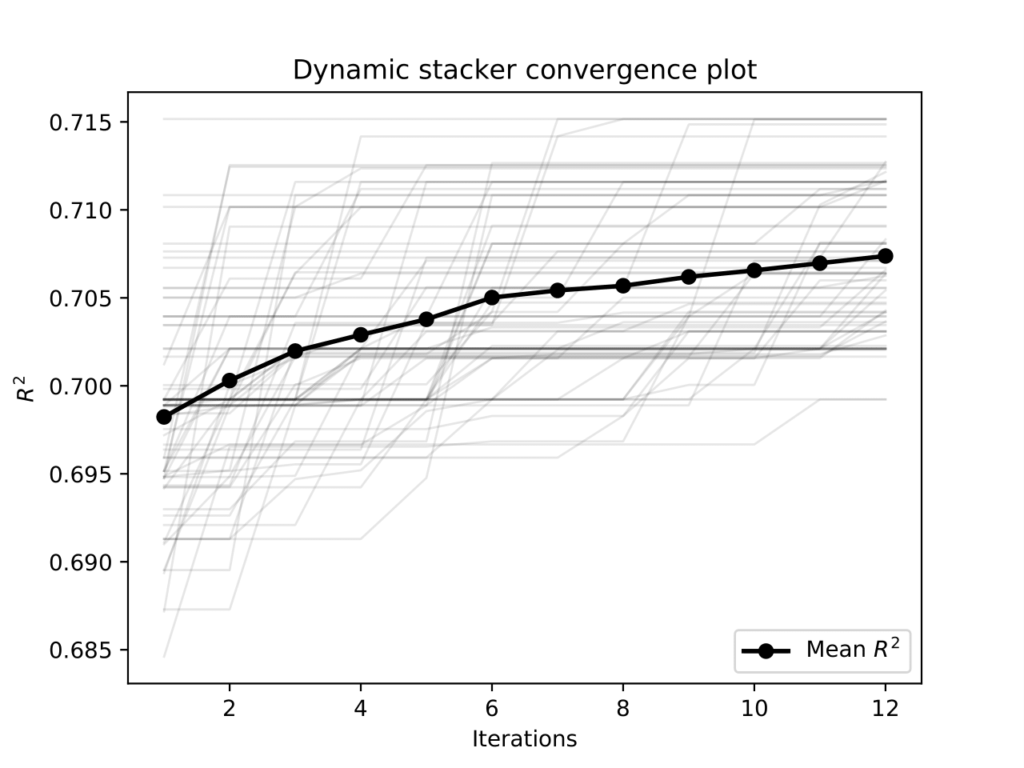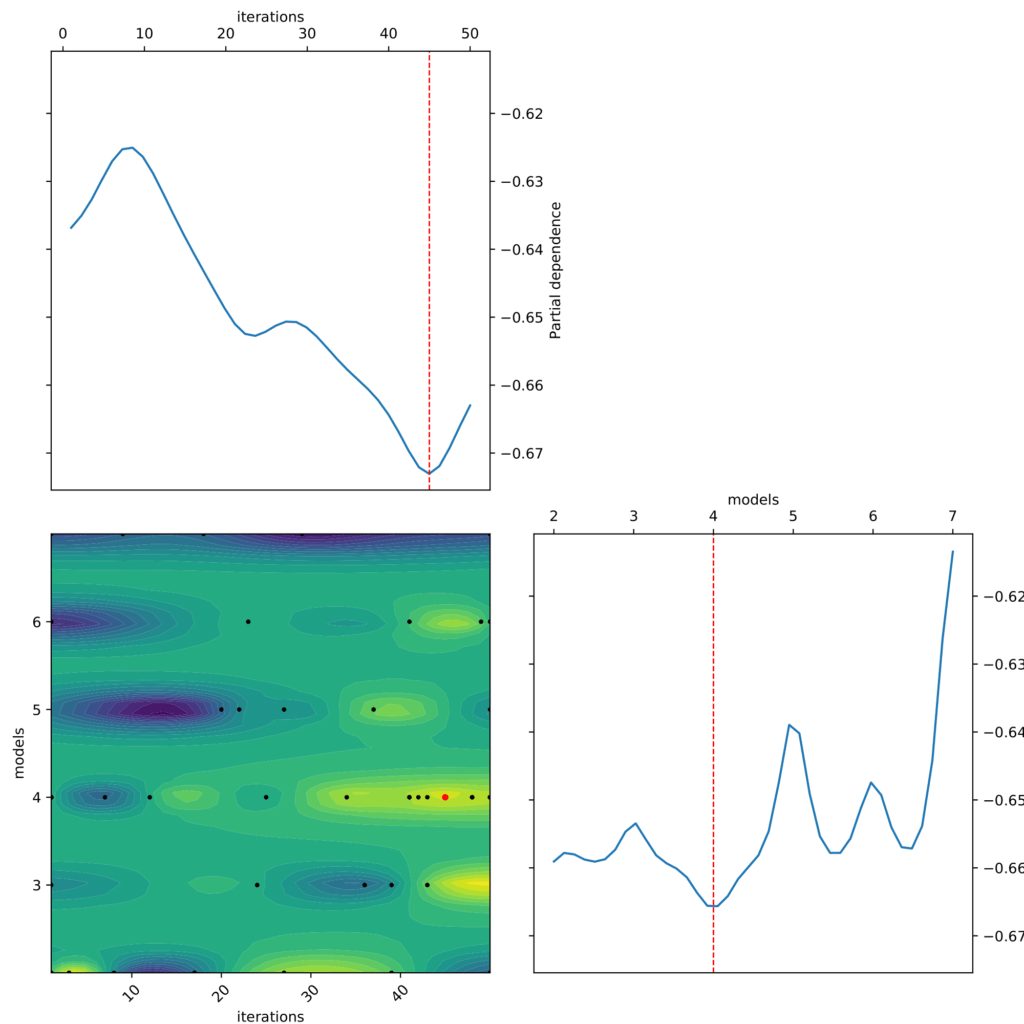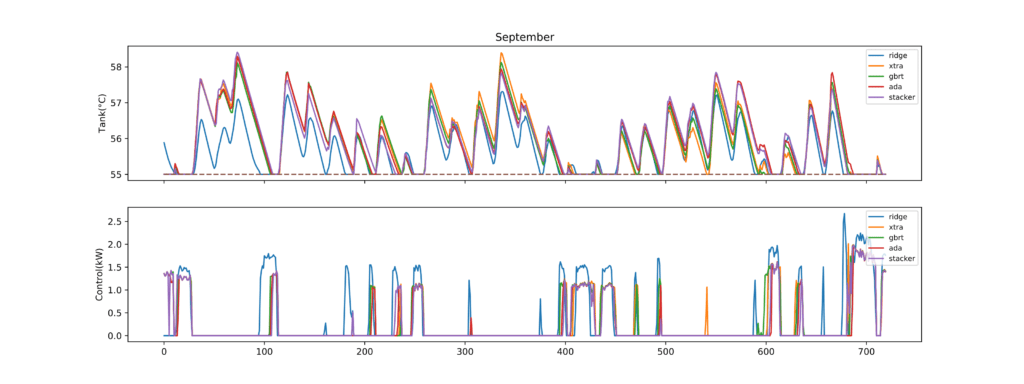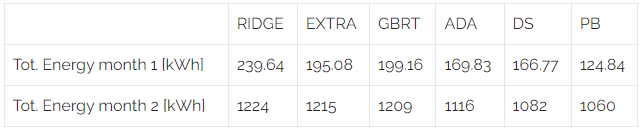## Optimizing models and controllers for distributed energy resources

In Demand Response programs, utilities use rate increases, bill credits or other incentives to control demand on the electric grid during periods when electricity demand is threatened to outpace the electricity supply.

Demand Response programs often include rewards or penalties to encourage customer’s behavior change.

Utilities are helping customers, using these programs, to adjust their energy consumption during Demand Response events through the use of new control systems. Such systems use forecasts to predict energy production and consumption, and accordingly optimize them, minimizing costs.

The use of good prediction models, that provide quality forecasts used for predicting uncertain renewable energy sources, can have a great impact on the controller performances in predictive control, providing extra safety and earnings for the utilities and energy distributors.

Unfortunately, to fine tune the prediction models’ parameters is often difficult, and requires expert experience. Therefore there is an interest to develop, in demand response, approaches that can optimize the performance of a set of given models.

In this article, we address this problem through the framework of Bayesian optimization, presenting a forward selection strategy that, combined with ensemble stacking allows

to improve the quality of the model and its predictions.

Through a case study of a residential family house, equipped with a heat pump and a solar collector, we show how our ensemble strategy, coupled with a model predictive controller, reduces the energy consumptions when compared with other machine learning prediction models. We also show that the proposed algorithm outperforms several different machine learning models in terms of prediction accuracy.

Finally, we discuss how better prediction accuracy from dynamic model stacking improves the

controller schedule, resulting in improved energy consumption.

## Problem

Distributed energy resources (DERs), such as photovoltaic and solar systems, make overall system control and operations very challenging, given high uncertainty due to high penetration of renewable energies.

To be able to make use of the distributed energy resources flexibility appropriately in real applications there is an increasing need for solving optimization problems that include the forecasts of disturbances such as solar radiation and wind speed.

Model Predictive Control (MPC) is an ideal framework to tackle this type of constrained problems. The idea behind MPC is to exploit a model of a process in combination with the disturbance forecasts of the system to predict its future evolution, and to compute control actions by optimizing a cost function dependent on these predictions. However, the performance of such an approach depends on the accuracy of the forecasts which have an inherent uncertainty.

We developed a forward selection algorithm based on ensemble stacking , that dynamically combines several different prediction models to improve the overall MPC performances.

## Ensemble Stacking

Stacking or stacked generalization, is a procedure of ensemble learning where a two-level model is trained on the output of a collection of base models.

In k-fold cross-validation, the data set is divided into k-subsets, and the holdout method is repeated k-times. Each time, one of the k-subsets is used as the test set and the other k − 1 subsets are put together to form a training set. Then the average error across all k-trials is computed.

Finding the best models for the ensemble requires fitting all the possible combinations of

models, which is a very expensive procedure. In our forward model selection procedure we use Bayesian optimization with Gaussian processes as prior functions to find the best models to add to the ensemble.

Figure 1 shows a diagram that illustrates how the data is divided for the stacking algorithm. The ensemble stacking procedure is summarized as follows: the total data set is split into two disjoint sets (train and test) using cross validation. Each base model is fitted on the first part (train) and predictions from all these base models are calculated on the second part (holdout), as illustrated in Figure 1. The inputs used to fit a higher level model called meta-model, are the predictions from the previous step (called out-of-folds predictions), and the output is the target variable Y. Finally, the stacking algorithm computes an average of the predictions for all base models on the test data and uses it as input on which, the final prediction is done by the meta-model.

Now let’s quickly review the general Bayesian optimization approach, before discussing our contributions. What makes Bayesian optimization appealing is that it constructs a probabilistic model with the observations  θ of a generic function f(θ), and then exploits this model to make decisions about where to evaluate f(θ), while integrating out uncertainty.

This procedure can find the minimum of difficult non-convex functions. The information available from previous evaluations of f is used instead of relying on a local gradient and Hessian approximations. As the number of observations θ performed increases, improving the posterior distribution, the algorithm becomes more certain of which regions in parameter space are worth exploring.

The two most important decisions to be made when performing Bayesian optimization are: the selection of a prior and the choice of an acquisition function, which is used to construct a utility function from the model posterior, allowing us to determine the next point to evaluate.

We can now define a forward selection algorithm to dynamically apply stacking to a subset of models of size M, given a set of models Ψm ∈ M ⊆ J , m = 1, . . . , M, that minimize a loss function f computed between the predictions of the stacked ensemble and the observed time series that we want to predict.

To select the best candidate model Ω we create a diverse set of base models Ψj using different algorithms. The base models parameters are initialized with a randomized grid search. After having initialized the models in J , we select M and γ by applying Bayesian optimization to minimize a loss function. Finally we select the best performing model based on a metric, such as R-squared.

## Experiments

In this section we perform numerical experiments to analyze the performances of the dynamic stacking algorithm combined with a model predictive controller. We compare the dynamic stacking algorithm with other machine learning algorithms, using two performance metrics:

• performance bound (PB)
• total power consumed.

The PB, is defined as optimal control with perfect information, which in our case will be

an MPC controller with perfect predictions, which is used as a benchmark. Perfect predictions means that the model is able to predict future values with zero error (zero residuals).

The experiments are performed using data collected from an experimental system located in a family house with a 3kW heating system and a solar collector.

We select the base and meta models from a pool of 35 models obtained setting different parameters from different algorithms including: ridge regression (RIDGE), extremely randomized trees (EXTRA), ada boost (ADA), Gradient Boosted Regression Trees (GBRT). We set the parameters of the Bayesian optimization iterations to 12 and maximum 30 iterations for random sampling the loss function. All simulations were carried out for a total of two monthse, covering all the data available.

## Results

We can now analyze how the MPC, defined before, performs when combined with the dynamic stacker (DS). First we identify the ensemble hyper-parameters combination (iterations and number of models in the ensemble) running the dynamic stacking algorithm.

In Figure 2 we show the algorithm improvement on the loss function  over 500 simulated experiments. The algorithm improves the initial R2 metric, on the training set, from 0.67 to 0.715.Figure 2. Convergence plot maximizing the R2 metric between the predictedand the real values over the training data set using dynamic stacking for 500different experiments. The grey lines represent each single experiment, whilethe dotted line is the mean per iteration.

Figure 3 illustrates the Gaussian Process predicted mean for one of the experiments. The minimum is found, with Bayesian optimization, for 44 iterations (γ) and 4 models (M). During the iterations the Bayesian algorithm balances its needs of exploration and exploitation taking into account its knowledge about the loss function.Figure 3. Contour lines of the loss function for one of the experiments. In redthe minimum is found for 44 iterations γ and 4 models M.

At each step a Gaussian Process is fitted to the known samples (previously explored points), and the posterior distribution, used to determine the next point that should be explored. This procedure allows to search quickly, a highly dimensional space, adequate models for predicting signals produced or consumed by DERs (e.g. electricity loads). This process is designed to minimize the number of steps required to find a combination of models for the ensemble. After having identified the hyper-parameters of the dynamic stacker, we can calculate the prediction performances (in terms of R2 ), over the shown in Table I.

After estimating the Dynamic Stacking ensemble, we simulate the MPC loop, for a total of two months. Figure 5 shows data from one of the simulations, illustrating the behavior of the same MPC algorithm with different predictions coming from different models: the dynamic stacker provides better forecasts and allows the MPC to produce a schedule that uses less heating power.Figure 5. Data illustrating the behavior of the same MPC algorithm with different predictions coming from different models, usingdata collected in the month of September.

In Table II, the controller performances are compared, in detail, using different prediction models. It is shown that the MPC with DS reduces the power consumption compared with the other cases.Table II. Total energy consumed by the MPC controller using different prediction models. PB is the performance bound with perfect predictions. The dynamic stacker (DS) is the one performing best.

If we compare these results with the different model performances (calculated on the test data set over 48 hours), from Table I, we observe a correlation between quality of the predictions and controller performances: a better prediction model is likely to improve controller performances. Moreover, a particular issue in the optimization process is that the prior is absolutely critical. Gaussian processes might not always be the best option. In cases where there is few information about the objective function, either strong assumptions are made without certainty that they hold, or a weak prior must be used. Additionally, it is often unclear how to handle the trade-off between exploration and exploitation in the acquisition function: if we explore too much many iterations could be wasted, without any improvement. When the dimensionality of the problem grows, these issues increase: more dimensions require a higher number of samples to cover the space. In a high multidimensional space, it might be necessary to optimize some dimensions individually or introduce a penalty. Finally, it is very important to have a good amount of performing models that have uncorrelated predictions: averaging uncorrelated predictions is a way of excluding models that express similar information. In order to choose a better model pool it is a good practice to calculate the Pearson’s correlation factor between each model prediction and select models that are less correlated.